Next: Time-Independent Perturbation Theory Up: Addition of Angular Momentum Previous: Calculation of Clebsch-Gordon Coefficients

# Exercises

1. Calculate the Clebsch-Gordon coefficients for adding spin one-half to spin one.

2. An electron in a hydrogen atom occupies the combined spin and position state whose spinor-wavefunction is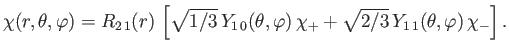Here,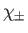are the eigenstates of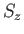corresponding to the eigenvalues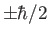, respectively, and,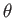,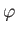are conventional spherical coordinates.
1. What values would a measurement of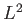yield, and with what probabilities?
2. Same for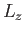.
3. Same for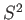.
4. Same for.
5. Same for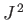.
6. Same for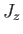.
7. What is the probability density for finding the electron at,,?
8. What is the probability density for finding the electron in the spin-up state (with respect to the-axis) at radius?


3. In a low energy neutron-proton system (with zero orbital angular momentum) the potential energy is given by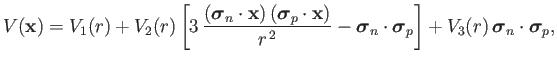where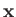is the vector connecting the two particles,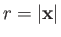,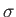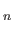denotes the vector of the Pauli matrices of the neutron, and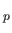denotes the vector of the Pauli matrices of the proton. Calculate the potential energy for the neutron-proton system:
1. In the spin singlet (i.e., spin zero) state.
2. In the spin triplet (i.e., spin one) state.
[Hint: Calculate the expectation value of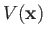with respect to the overall spin state.] 

4. Consider two electrons in a spin singlet (i.e., spin zero) state.
1. If a measurement of the spin of one of the electrons shows that it is in the state with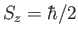, what is the probability that a measurement of the-component of the spin of the other electron yields?
2. If a measurement of the spin of one of the electrons shows that it is in the state with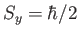, what is the probability that a measurement of the-component of the spin of the other electron yields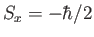?
3. Finally, if electron 1 is in a spin state described by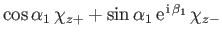, and electron 2 is in a spin state described by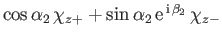, what is the probability that the two-electron spin state is a triplet (i.e., spin one) state? Here,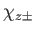are the eigenstates ofcorresponding to the eigenvalues,, respectively, for the electron in question.Next: Time-Independent Perturbation Theory Up: Addition of Angular Momentum Previous: Calculation of Clebsch-Gordon Coefficients
Richard Fitzpatrick 2016-01-22